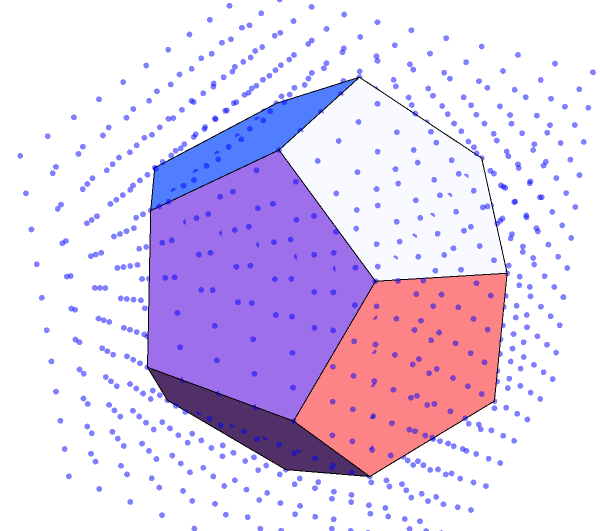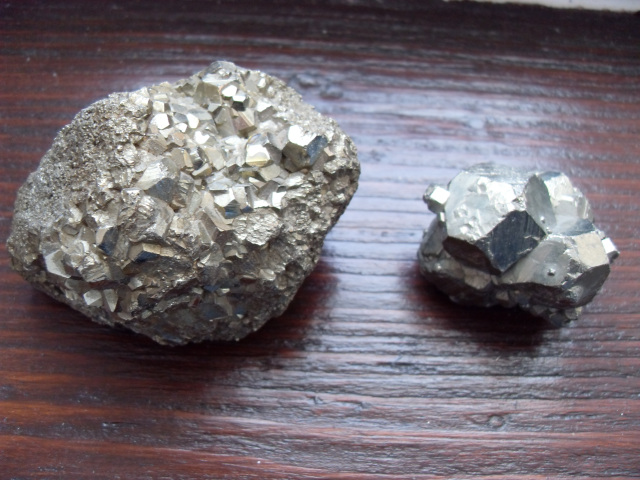# Rational approximations to Platonic solids

It is straightforward to position a cube such that its vertices are all integer coordinates. For instance, we could choose its eight vertices to be (±1, ±1, ±1). Similarly, the vertices of a regular octahedron can be positioned at cyclic permutations of (0, 0, ±1). The tetrahedron, being a subset of the cube, can also be realised exactly with integer coordinates.

What about the dodecahedron and icosahedron? If we could position all the vertices at integer coordinates, then all distances must be square-roots of integers (by Pythagoras). However, since φ² is irrational, that precludes the possibility of two lengths in the ratio 1 : φ (necessary for regular pentagons to exist). Hence, it is impossible to give all vertices of a regular dodecahedron rational coordinates.

This suggests the problem of finding best rational approximations to the dodecahedron and icosahedron. For the dodecahedron, James Buddenhagen gave a set of coordinates for a pyritohedron which differs from regularity by a microscopic amount. Relaxing the condition that the faces must be exactly planar, a nice sequence of good approximations to the regular dodecahedron is obtained by taking all even permutations and sign changes of (x, z, 0) and (y, y, y), where x < y < z are three consecutive Fibonacci numbers.With the icosahedron, the faces are triangular so there is no need to worry about planarity. In this case, we only need to take two consecutive Fibonacci numbers; the icosahedron is the convex hull of all even permutations of (±x, ±y, 0). I conjecture that this gives the best rational approximations to the regular icosahedron, where ‘best’ is defined as ‘least variance (normalised by dividing by the squared mean) of edge length’. It is optimal amongst all icosahedra with pyritohedral symmetry, at least, since the best rational approximations to φ are ratios between consecutive Fibonacci numbers.

### Etymology of ‘pyritohedron’

Pyritohedra are so named because they occur naturally in crystals of iron pyrite (a.k.a. fools’ gold). I happen to have a couple of iron pyrite crystals I photographed years ago:The larger sample clearly features partial examples of what appear to be regular dodecahedra, but are actually irregular as a consequence of the crystallographic restriction theorem. The smaller sample appears to feature truncated octahedra instead, and the crystallographic restriction theorem does not preclude their uniformity. Indeed, as an easy exercise for the reader, find integer coordinates for the 24 vertices of a truncated octahedron such that all edge lengths are equal. John Baez remarked that in addition to dodecahedra with pyritohedral symmetry, their geometric duals (irregular icosahedra) also occur in crystals of iron pyrite.

Now for a historical digression: It has been suggested that the Pythagoreans (who definitely knew of the dodecahedron, or ‘sphere of twelve pentagons’) may have discovered the regular dodecahedron by idealising the pyritohedra found in nature. On the other hand, when Thaetetus exhaustively proved that there are only five Platonic solids, he used purely theoretical methods similar to the idea of a Schläfli symbol. Tim Hutton independently repeated the same lines of reasoning, finding the planar and hyperbolic tilings in the process.

There is a subtle aspect to the crystallographic restriction theorem: it only applies to true crystals with translational symmetry. In the latter half of the twentieth century, quasicrystals were discovered; these are three-dimensional generalisations of Penrose tilings, usually found in metal alloys. One particular quasicrystal has perfect icosahedral symmetry. If we lived in four-dimensional space, we could also get quasicrystals with H4 symmetry (the largest polychoral point group), based on a tessellation by Viet Elser and Neil Sloane.

This entry was posted in Uncategorized. Bookmark the permalink.

### 5 Responses to Rational approximations to Platonic solids

1.authorized appliance repair says:

I was recommended this website via my cousin. I am now not certain whether this publish is written via him as nobody else realize such special about my problem.

You’re incredible! Thanks!

•EMF says:

SPAM SPAM SPAM SPAM

2.Daniel Sebald says:

Do the great dodecahedron and small stellated dodecahedron have rational isomorphs?

3.Gerry Myerson says:

https://mathoverflow.net/questions/452847/can-an-icosahedron-contain-a-rational-point-on-each-face asks whether a regular icosahedron can contain a rational point on each face.